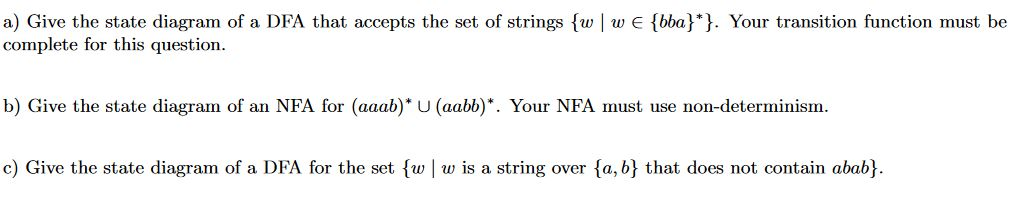# a) Give the state diagram of a DFA that accepts the set of strings fw wE (bba}*}. Your transition function must be complete for this question b) Give the state diagram of an NFA for (aaab)* U (aabb)*. Your NFA must use non-determinism. c) Give the state diagram of a DFA for the set fw w is a string over fa, b} that does not contain abab}

Question

Please explain how you solve the follwing problem:help_outlineImage Transcriptionclosea) Give the state diagram of a DFA that accepts the set of strings fw wE (bba}*}. Your transition function must be complete for this question b) Give the state diagram of an NFA for (aaab)* U (aabb)*. Your NFA must use non-determinism. c) Give the state diagram of a DFA for the set fw w is a string over fa, b} that does not contain abab} fullscreen

1 Rating

### Want to see the step-by-step answer?